# Computer Number Systems and its types

## What are the number systems in Computer?

Number systems are the technique to represent numbers in the computer system architecture, every value that you are saving or getting into/from computer memory has a defined number system.

Computer architecture supports following number systems.

• Binary number system
• Octal number system
• Decimal number system

### 1) Binary Number System

A Binary number system has only two digits that are 0 and 1. Every number (value) represents with 0 and 1 in this number system. The base of binary number system is 2, because it has only two digits.

### 2) Octal number system

Octal number system has only eight (8) digits from 0 to 7. Every number (value) represents with 0,1,2,3,4,5,6 and 7 in this number system. The base of octal number system is 8, because it has only 8 digits.

### 3) Decimal number system

Decimal number system has only ten (10) digits from 0 to 9. Every number (value) represents with 0,1,2,3,4,5,6, 7,8 and 9 in this number system. The base of decimal number system is 10, because it has only 10 digits.

A Hexadecimal number system has sixteen (16) alphanumeric values from 0 to 9 and A to F. Every number (value) represents with 0,1,2,3,4,5,6, 7,8,9,A,B,C,D,E and F in this number system. The base of hexadecimal number system is 16, because it has 16 alphanumeric values. Here A is 10, B is 11, C is 12, D is 13, E is 14 and F is 15.

Table of the Numbers Systems with Base, Used Digits, Representation, C language representation:

 Number system Base Used digits Example C Language assignment Binary 2 0,1 (11110000)2 int val=0b11110000; Octal 8 0,1,2,3,4,5,6,7 (360)8 int val=0360; Decimal 10 0,1,2,3,4,5,6,7,8,9 (240)10 int val=240; Hexadecimal 16 0,1,2,3,4,5,6,7,8,9,A,B,C,D,E,F (F0)16 int val=0xF0;

## Number System Conversions

There are three types of conversion:
• Decimal Number System to Other Base
[for example: Decimal Number System to Binary Number System]
• Other Base to Decimal Number System
[for example: Binary Number System to Decimal Number System]
• Other Base to Other Base
[for example: Binary Number System to Hexadecimal Number System]

### Decimal Number System to Other Base

To convert Number system from Decimal Number System to Any Other Base is quite easy; you have to follow just two steps:
A) Divide the Number (Decimal Number) by the base of target base system (in which you want to convert the number: Binary (2), octal (8) and Hexadecimal (16)).
B) Write the remainder from step 1 as a Least Signification Bit (LSB) to Step last as a Most Significant Bit (MSB).

 Decimal to Binary Conversion Result Decimal Number is : (12345)10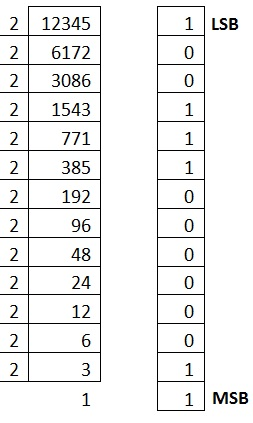Binary Number is (11000000111001)2

 Decimal to Octal Conversion Result Decimal Number is : (12345)10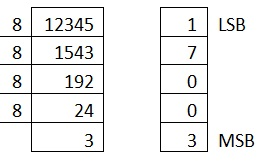Octal Number is (30071)8

 Decimal to Hexadecimal Conversion Result Example 1 Decimal Number is : (12345)10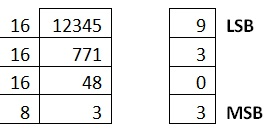Hexadecimal Number is (3039)16 Example 2 Decimal Number is : (725)10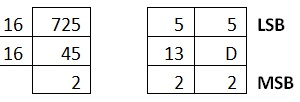Hexadecimal Number is (2D5)16 Convert 10, 11, 12, 13, 14, 15 to its equivalent... A, B, C, D, E, F

### Other Base System to Decimal Number Base

To convert Number System from Any Other Base System to Decimal Number System, you have to follow just three steps:
A) Determine the base value of source Number System (that you want to convert), and also determine the position of digits from LSB (first digit’s position – 0, second digit’s position – 1 and so on).
B) Multiply each digit with its corresponding multiplication of position value and Base of Source Number System’s Base.
C) Add the resulted value in step-B.

Explanation regarding examples:
Below given exams contains the following rows:
A) Row 1 contains the DIGITs of number (that is going to be converted).
B) Row 2 contains the POSITION of each digit in the number system.
C) Row 3 contains the multiplication: DIGIT* BASE^POSITION.
D) Row 4 contains the calculated result of step C.
E) And then add each value of step D, resulted value is the Decimal Number.

 Binary to Decimal Conversion Binary Number is : (11000000111001)2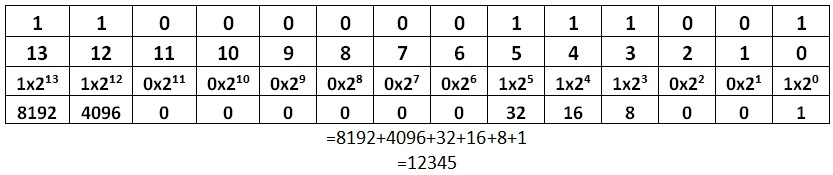Octal to Decimal Conversion Result Octal Number is : (30071)8 ```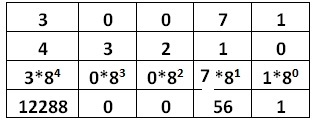``` =12288+0+0+56+1 =12345 Decimal Number is: (12345)10

 Hexadecimal to Decimal Conversion Result Hexadecimal Number is : (2D5)16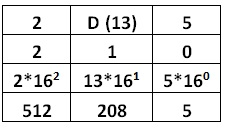=512+208+5 =725 Decimal Number is: (725)10

Recommended posts

Top MCQs

Languages: » C » C++ » C++ STL » Java » Data Structure » C#.Net » Android » Kotlin » SQL
Web Technologies: » PHP » Python » JavaScript » CSS » Ajax » Node.js » Web programming/HTML
Solved programs: » C » C++ » DS » Java » C#
Aptitude que. & ans.: » C » C++ » Java » DBMS
Interview que. & ans.: » C » Embedded C » Java » SEO » HR
CS Subjects: » CS Basics » O.S. » Networks » DBMS » Embedded Systems » Cloud Computing
» Machine learning » CS Organizations » Linux » DOS
More: » Articles » Puzzles » News/Updates